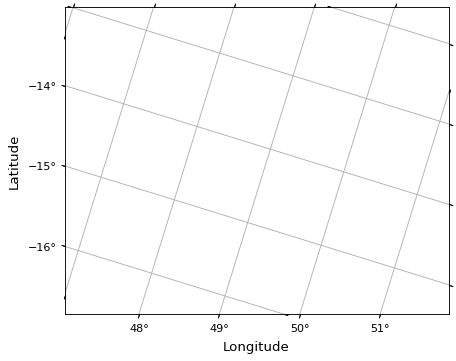# Initializing WCSAxes with custom transforms¶

In Initializing axes with world coordinates, we saw how to make plots using `WCS` objects. However, the `WCSAxes` class can also be initialized with more general transformations that don’t have to be represented by the `WCS` class. Instead, you can initialize `WCSAxes` using a Matplotlib `Transform` object and a dictionary (`coord_meta`) that provides metadata on how to interpret the transformation.

The `Transform` should represent the conversion from pixel to world coordinates, and should have `input_dims=2` and can have `output_dims` set to any positive integer. In addition, `has_inverse` should be set to `True` and the `inverted` method should be implemented.

The `coord_meta` dictionary should include the following keys:

• `name`: an iterable of strings giving the names for each dimension

• `type`: an iterable of strings that should be either `'longitude'`, `'latitude'`, or `'scalar'` (for anything that isn’t a longitude or latitude).

• `wrap`: an iterable of values which indicate for longitudes at which angle (in degrees) to wrap the coordinates. This should be `None` unless `type` is `'longitude'`.

• `unit`: an iterable of `Unit` objects giving the units of the world coordinates returned by the `Transform`.

• `format_unit`: an iterable of `Unit` objects giving the units to use for the formatting of the labels. These can be set to `None` to default to the units given in `unit`, but can be set for example if the `Transform` returns values in degrees and you want the labels to be formatted in hours.

In addition the `coord_meta` can optionally include the following keys:

• `default_axislabel_position`: an iterable of strings giving for each world coordinates the spine of the frame on which to show the axis label for the coordinate. Each string should be such that it could be used as input to `set_axislabel_position()`.

• `default_ticklabel_position`: an iterable of strings giving for each world coordinates the spine of the frame on which to show the tick labels for the coordinate. Each string should be such that it could be used as input to `set_ticklabel_position()`.

• `default_ticks_position`: an iterable of strings giving for each world coordinates the spine of the frame on which to show the ticks for the coordinate. Each string should be such that it could be used as input to `set_ticks_position()`.

The following example illustrates a custom projection using a transform and `coord_meta`:

```from astropy import units as u
import matplotlib.pyplot as plt
from matplotlib.transforms import Affine2D
from astropy.visualization.wcsaxes import WCSAxes

# Set up an affine transformation
transform = Affine2D()
transform.scale(0.01)
transform.translate(40, -30)

coord_meta = {}
coord_meta['name'] = 'lon', 'lat'
coord_meta['type'] = 'longitude', 'latitude'
coord_meta['wrap'] = 180, None
coord_meta['unit'] = u.deg, u.deg
coord_meta['format_unit'] = None, None

fig = plt.figure()
ax = WCSAxes(fig, [0.1, 0.1, 0.8, 0.8], aspect='equal',
transform=transform, coord_meta=coord_meta)